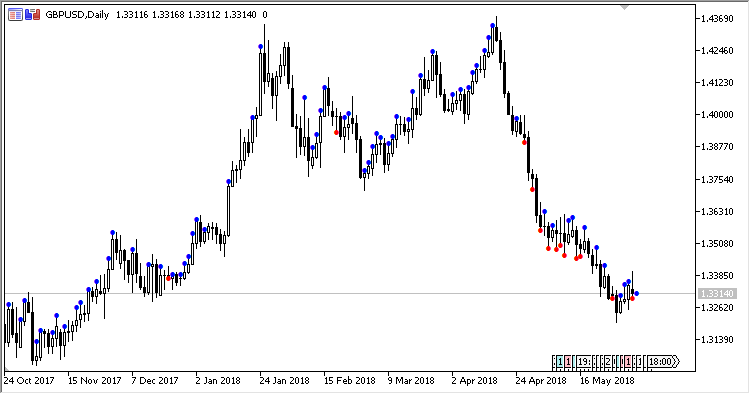Interesting script?
So post a link to it -
let others appraise it

You liked the script? Try it in the MetaTrader 5 terminalViews:
1315
Rating:
Published:
2018.07.09 11:05
Updated:
2018.09.17 10:57

The indicator is intended for roughly estimating the direction of the next candlestick, depending on exceeding the average candlestick range over a period of time.

If the range of the current candlestick exceeds at least double (to be defined in the parameters) of the average range of candlesticks over a period of time, the next candlestick can be in the same direction as the current one.

It has two parameters:

• Period - period for calculating the average range
• Multiplier - multiplier to calculate the threshold value

Calculation:

```If SoT > AVG * Multiplier, then
The next candlestick must have the same direction as the current one
```

where:

```SoT = ((Close - Open) / Volume) * 1000000000
AVG = MA(SoT, Period)
```Translated from Russian by MetaQuotes Software Corp.
Original code: https://www.mql5.com/ru/code/20990Reverse_MACD

An information-forecast MACD on the price chartRange_Contraction

Indicator of daily rangesTCI

Indicator Trend Confirmation IndexVolatilityMA

A moving average with a changeable calculation period.